Processing ......FreeComputerBooks.com Links to Free Computer, Mathematics, Technical Books all over the World

Introduction to Financial Mathematics: Concepts and Computational Methods
Top Free Programming Books 🌠 - 100% Free or Open Source!
• Title: Introduction to Financial Mathematics: Concepts and Computational Methods
• Author(s) Arash Fahim
• Publisher: Florida State University (2019); eBook (Creative Commons Licensed)
• Paperback: N/A
• eBook: PDF (290 pages, 3.10 MB)
• Language: English
• ASIN/ISBN-10: N/A
• ISBN-13: N/A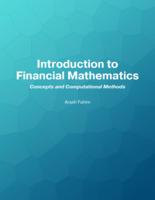Book Description

This text serves as a primer in financial mathematics with a focus on conceptual understanding of models and problem solving. It includes the mathematical background needed for risk management, such as probability theory, optimization, and the like.

The goal of the book is to expose the reader to a wide range of basic problems, some of which emphasize analytic ability, some requiring programming techniques and others focusing on statistical data analysis. In addition, it covers some areas which are outside the scope of mainstream financial mathematics textbooks.

For example, it presents marginal account setting by the CCP and systemic risk, and a brief overview of the model risk. Inline exercises and examples are included to help students prepare for exams on this book.

• N/A
Reviews, Ratings, and Recommendations: Related Book Categories: Read and Download Links:Similar Books:
•Financial Mathematics (C.H. Richardson, et al.)

Covering the theories of interest rates, with applications to the evaluation of cash flows, the pricing of fixed income securities and the management of bonds, this textbook also contains numerous examples and exercises of financial calculation.

•Mathematics of Economics and Business (Frank Werner, et al.)

For all students who wish to understand current economic and business literature, knowledge of mathematical methods has become a prerequisite. Clear and concise, with precise definitions and theorems, this book covers all the major topics required.

•Financial Numerical Recipes in C++: Applications in Finance

This book provides a good deal of useful examples and algorithms for people working within the field of finance, in C++. All the routines have been made to confirm to the new ISO/ANSI C++ standard, using namespaces and the standard template library.

•A Basic Course in the Theory of Interest and Derivatives Markets

This book is designed for an introductory course in the theory of interest and annuity. Each section contains the embedded examples with answer keys. It is suitable for a junior level course in the mathematics of finance.

•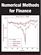Numerical Methods for Finance (Robert Nurnberg)

This book explores new and relevant numerical methods for the solution of practical problems in finance. It is one of the few books entirely devoted to numerical methods as applied to the financial field.

•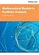Mathematical Models in Portfolio Analysis (Farida Kachapova)

This book explains portfolio modelling in financial mathematics as a consistent mathematical theory with all steps justified. The topics include mean-variance portfolio analysis and capital market theory. The book contains many examples with solutions.

•Analysis and Linear Algebra for Finance (Patrick Roger)

This book presents the elements of analysis and linear algebra used in financial models and in microeconomics. Functions of one and several variables and matrices are developed as well as vector spaces, linear mappings and optimization methods, etc.

•Mathematics for Finance: An Introduction to Financial Engineering

This book is an excellent introduction to Mathematical Finance. Armed with a knowledge of basic calculus and probability a student can use this book to learn about derivatives, interest rates and their term structure and portfolio management.

•Statistical Tools for Finance and Insurance (Pavel Cizek, et al)

Presents ready-to-use solutions, theoretical developments and method construction for many practical problems in quantitative finance and insurance, offers a unique combination of topics from which every market analyst and risk manager will benefit.

•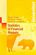Statistics of Financial Markets: An Introduction (Jürgen Franke)

The focus is both on fundamentals of mathematical finance and financial time series analysis and on applications to given problems of financial markets.

•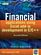Financial Applications using Excel Add-in in C/C++ (Steve Dalton)

This book is a must-buy book for any serious Excel developer, the only complete how-to guide and reference book for the creation of high performance add-ins for Excel in C and C++ for users in the finance industry.

Book Categories
 :All CategoriesTop Free BooksRecent BooksMiscellaneous BooksComputer EngineeringComputer LanguagesComputer ScienceData Science/DatabasesJava and Java EE (J2EE)Linux and UnixMathematicsMicrosoft and .NETMobile ComputingNetworking and CommunicationsSoftware EngineeringSpecial TopicsWeb Programming
Other Categories